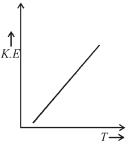Courses

# Kinetic Theory Of Gases MCQ Level – 1(Part - 1)

## 10 Questions MCQ Test Topic wise Tests for IIT JAM Physics | Kinetic Theory Of Gases MCQ Level – 1(Part - 1)

Description
This mock test of Kinetic Theory Of Gases MCQ Level – 1(Part - 1) for IIT JAM helps you for every IIT JAM entrance exam. This contains 10 Multiple Choice Questions for IIT JAM Kinetic Theory Of Gases MCQ Level – 1(Part - 1) (mcq) to study with solutions a complete question bank. The solved questions answers in this Kinetic Theory Of Gases MCQ Level – 1(Part - 1) quiz give you a good mix of easy questions and tough questions. IIT JAM students definitely take this Kinetic Theory Of Gases MCQ Level – 1(Part - 1) exercise for a better result in the exam. You can find other Kinetic Theory Of Gases MCQ Level – 1(Part - 1) extra questions, long questions & short questions for IIT JAM on EduRev as well by searching above.
QUESTION: 1

### At a constant pressure of the following graph, the one which represents the variation of the density of an ideal gas with the absolute temperature T, is Select one:

Solution:

We know the relation between Pressure and Density.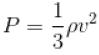when P is constant
pv= constant
or
dT = constant
The correct answer is: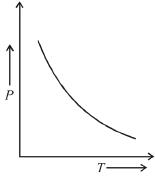QUESTION: 2

### Four molecules of a gas are having speed of 1, 4, 8 and 16 ms-1. The root mean square velocity of the gas molecules is Select one:

Solution:

Root mean square speed,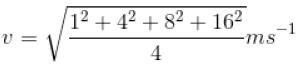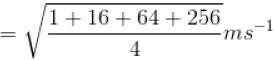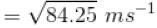= 9.2 ms-1
The correct answer is: 9.2 ms-1

QUESTION: 3

### Root mean square velocity of gas molecule is 300 ms-1. The r.m.s velocity of molecule of gas with twice the molecular weight and half absolute temperature is. Select one:

Solution:

The r.m.s. velocity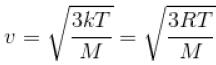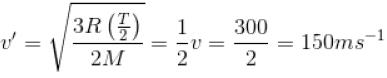The correct answer is: 150 ms-1

QUESTION: 4

The energy density u/V of an ideal gas is related to its pressure p as.
Select one:

Solution:

If u/V is energy density then from relation.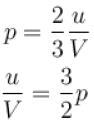The correct answer is: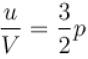QUESTION: 5

Arrange root mean square; most probable and average speeds in the order of increasing value.

Select one:

Solution: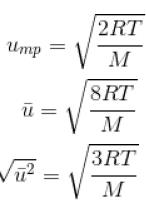Therefore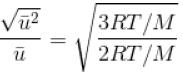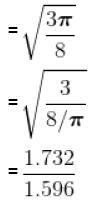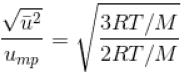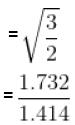Hence,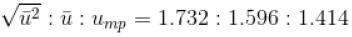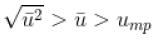The correct answer is: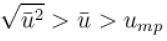QUESTION: 6

V versus T curves at constant pressure P1 and P2 for an ideal gas as shown in figure. Here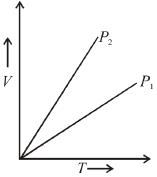Select one:

Solution:

From the ideal gas equation PV = nRT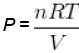or,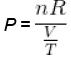But  V/T = Slope of the graph
So,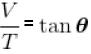∴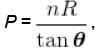where  tanθ is the slope of the graph
So, more the slope, lesser will be the pressure.
P1 > P2

QUESTION: 7

The density of air at a less of 105 Nm-2 is 1.2 kgm-3. Under these condition, the root mean square velocity of the air molecule in ms-1 is
Select one:

Solution:

Root mean square speed,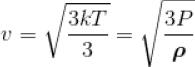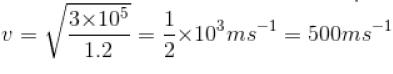QUESTION: 8

Formula for average speed of a gas having M molecular mass.
Select one:

Solution:
QUESTION: 9

For O2 gas molecule, the most probable speed at  temperature T is equal to 1.5 × 103 ms-1 , then temperature T is
Select one:

Solution:

We know,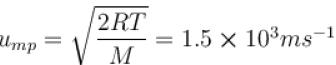Then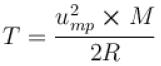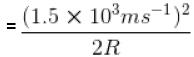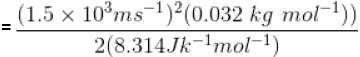= 4330K
The correct answer is: 4330 K

QUESTION: 10

Which of the following graphs best represents the relationship between absolute temperature of a gas and the average kinetic energy of the gas molecules?
Select one:

Solution:

We know that K.E. is directly proportional to temperature.
The correct answer is: